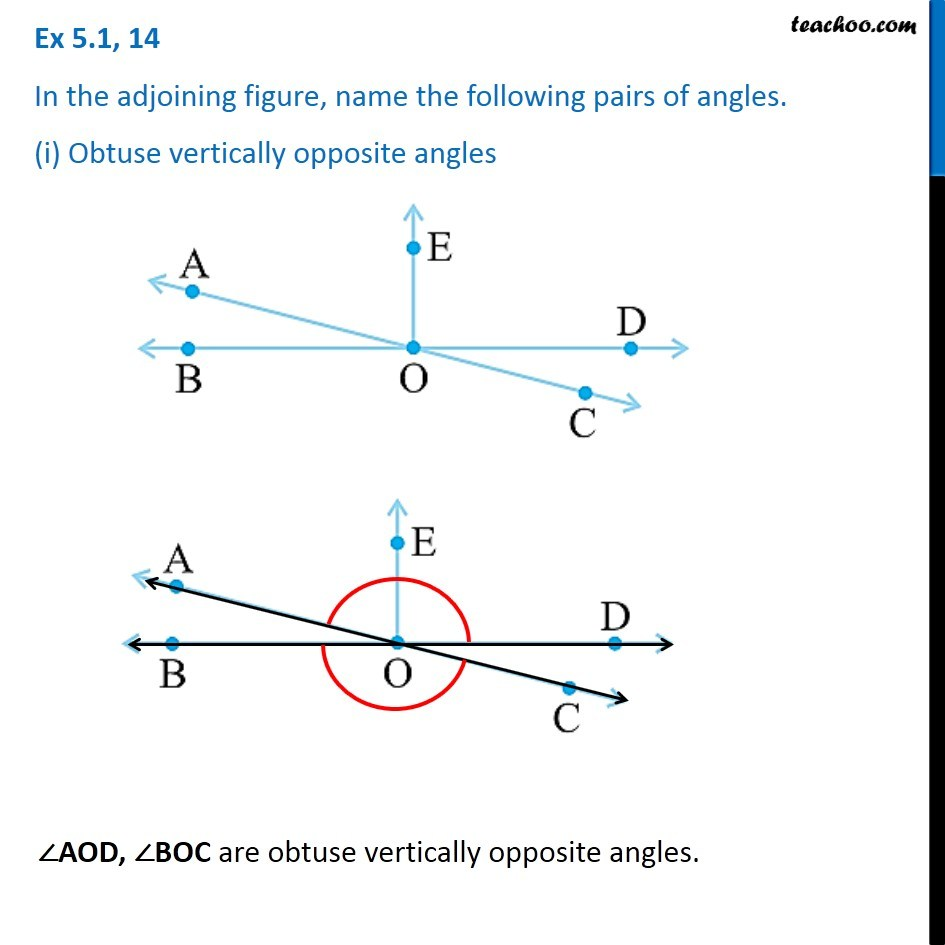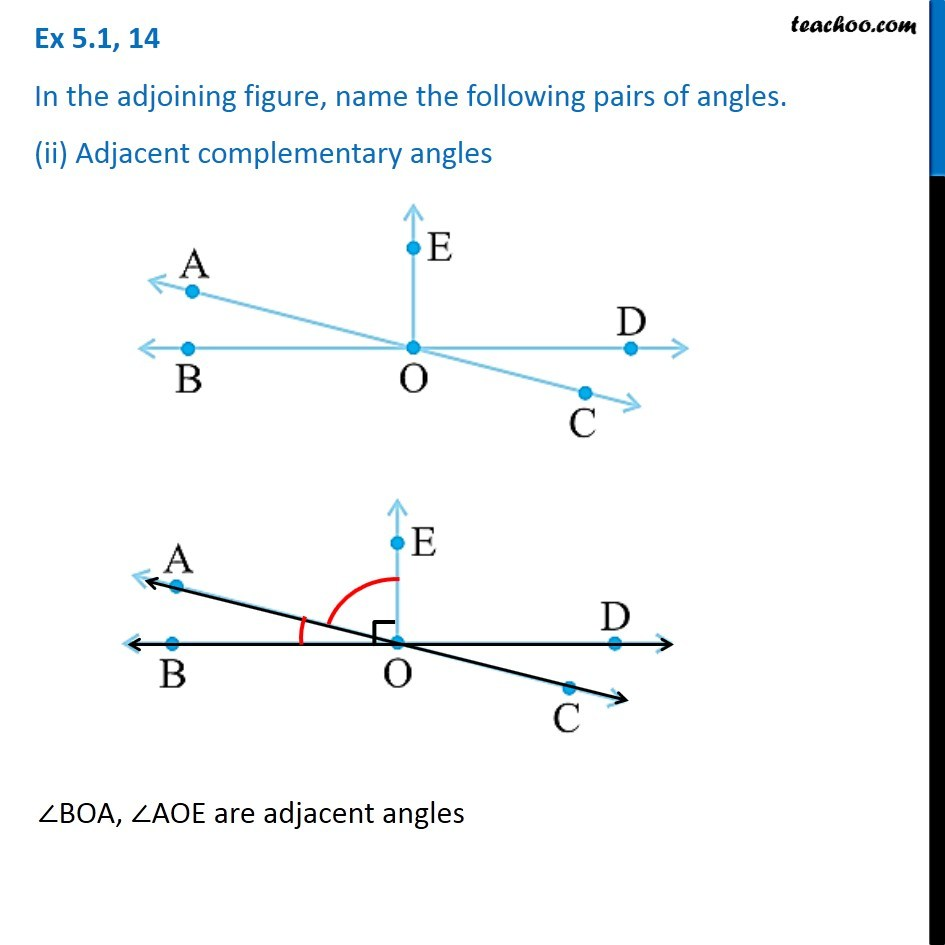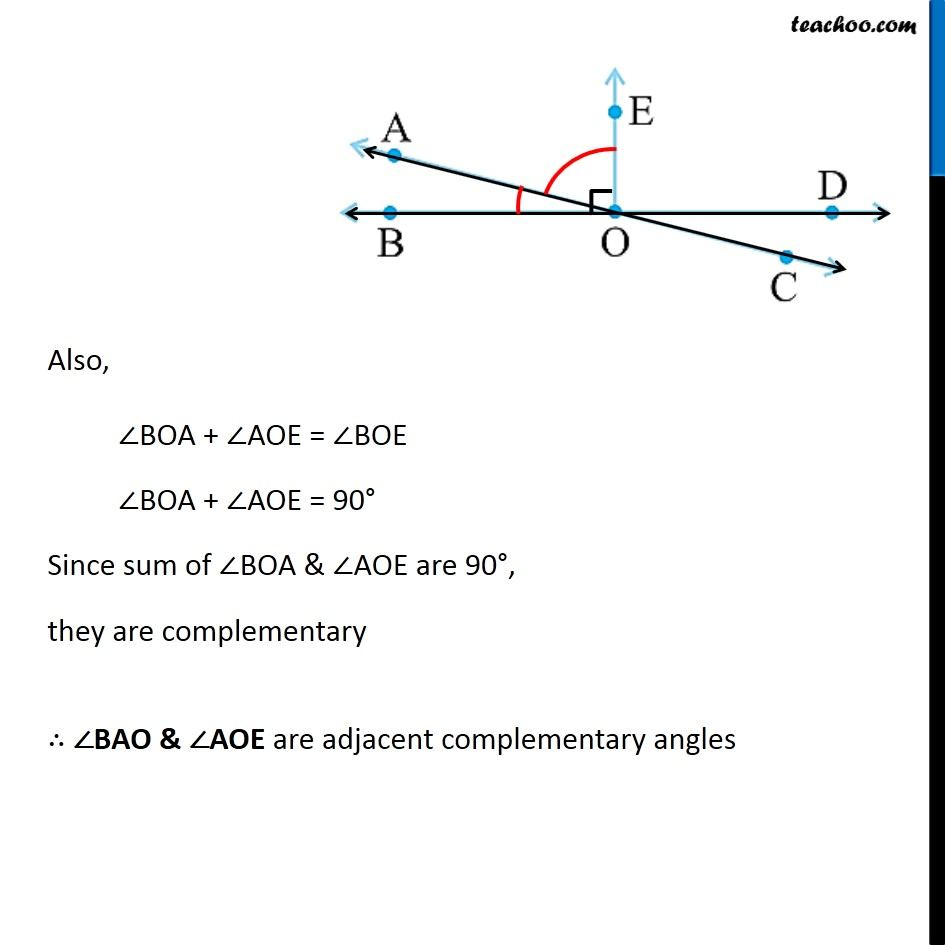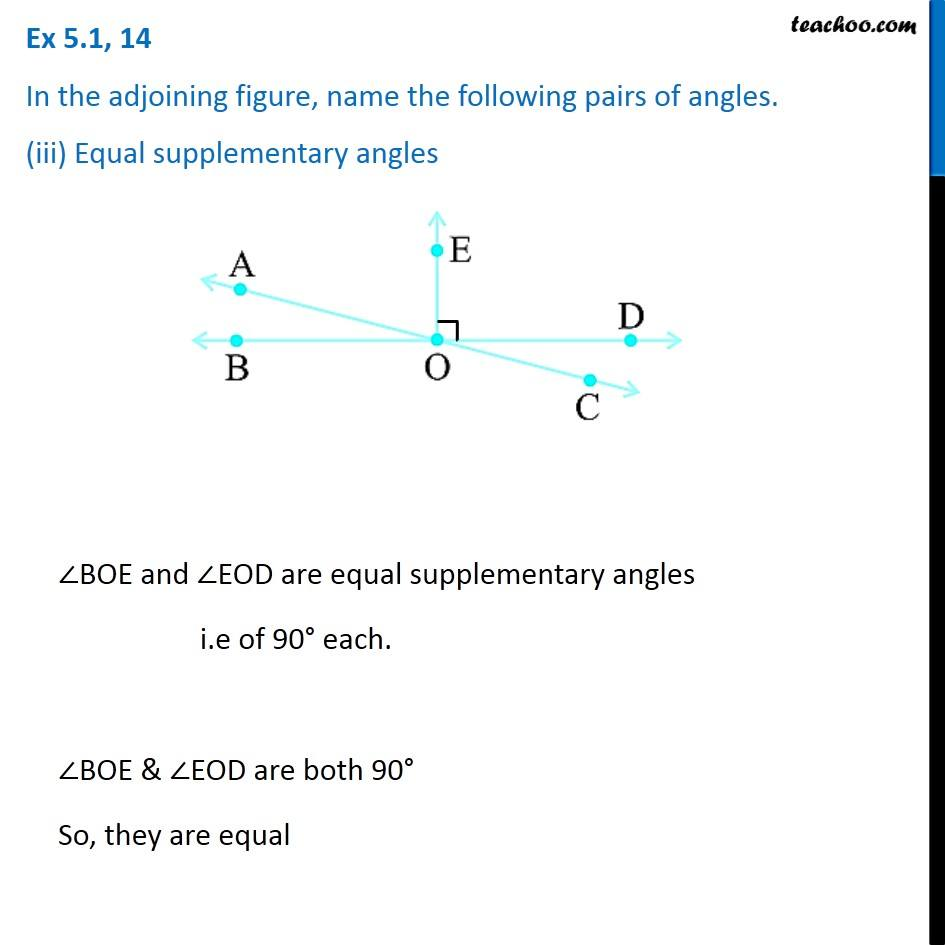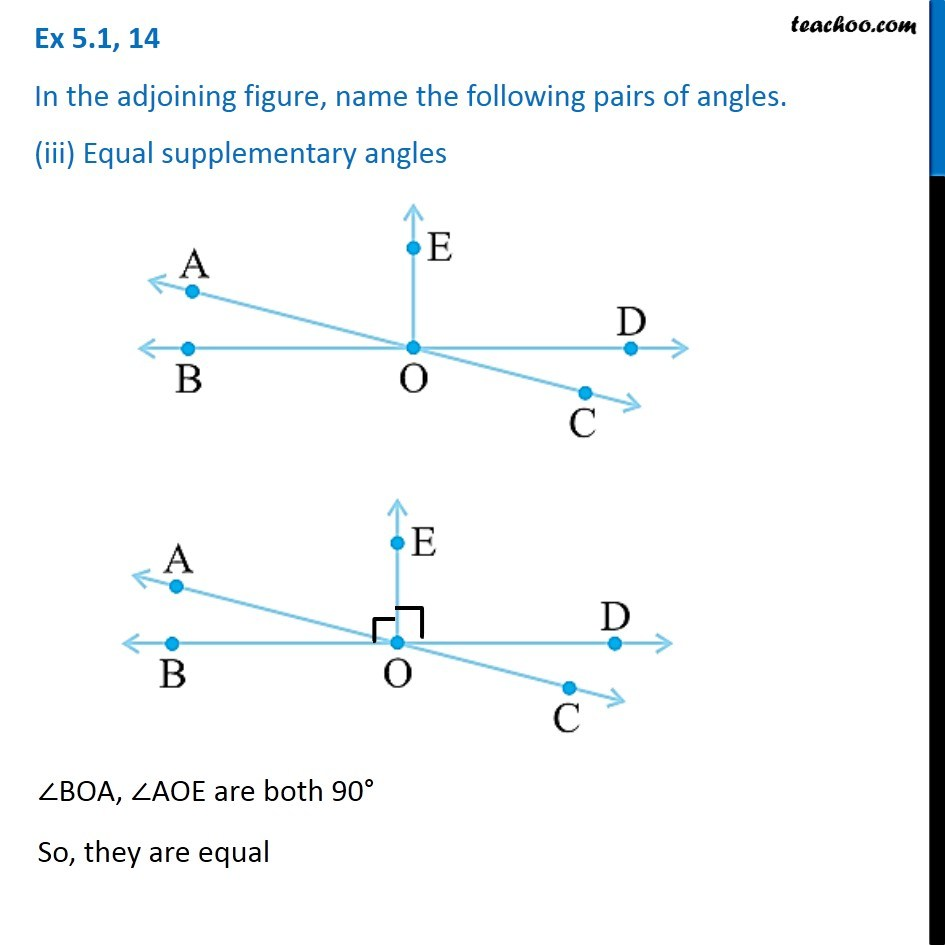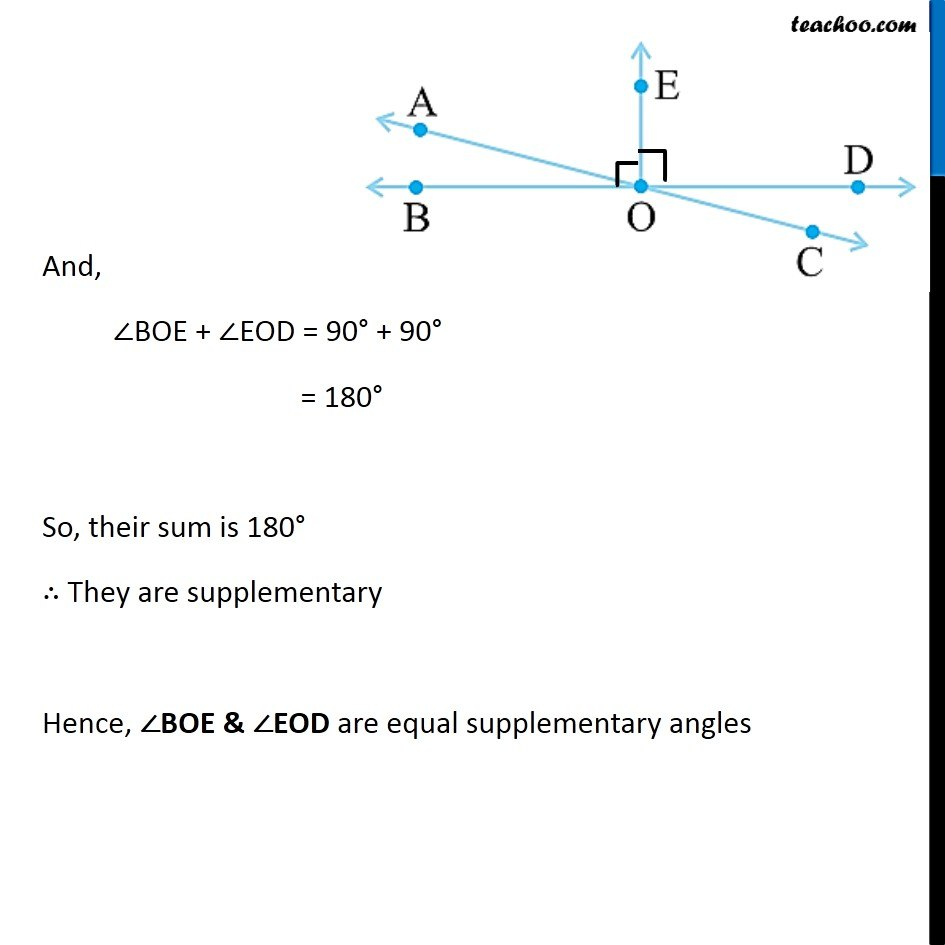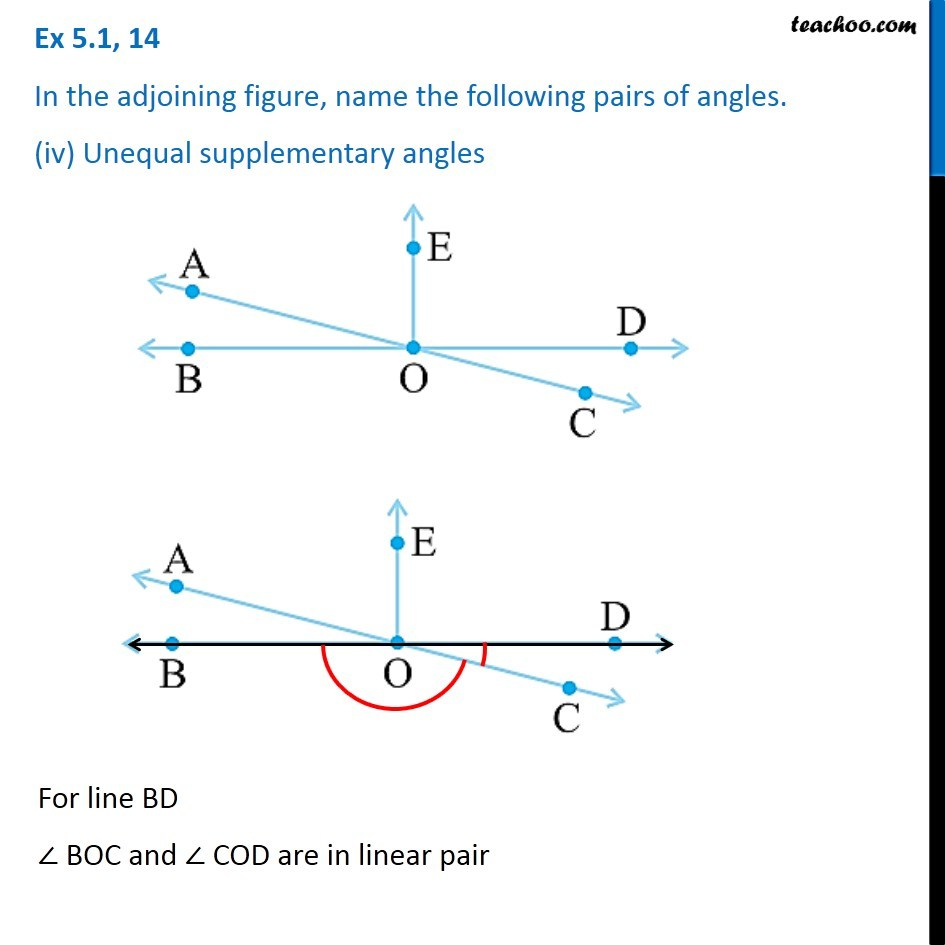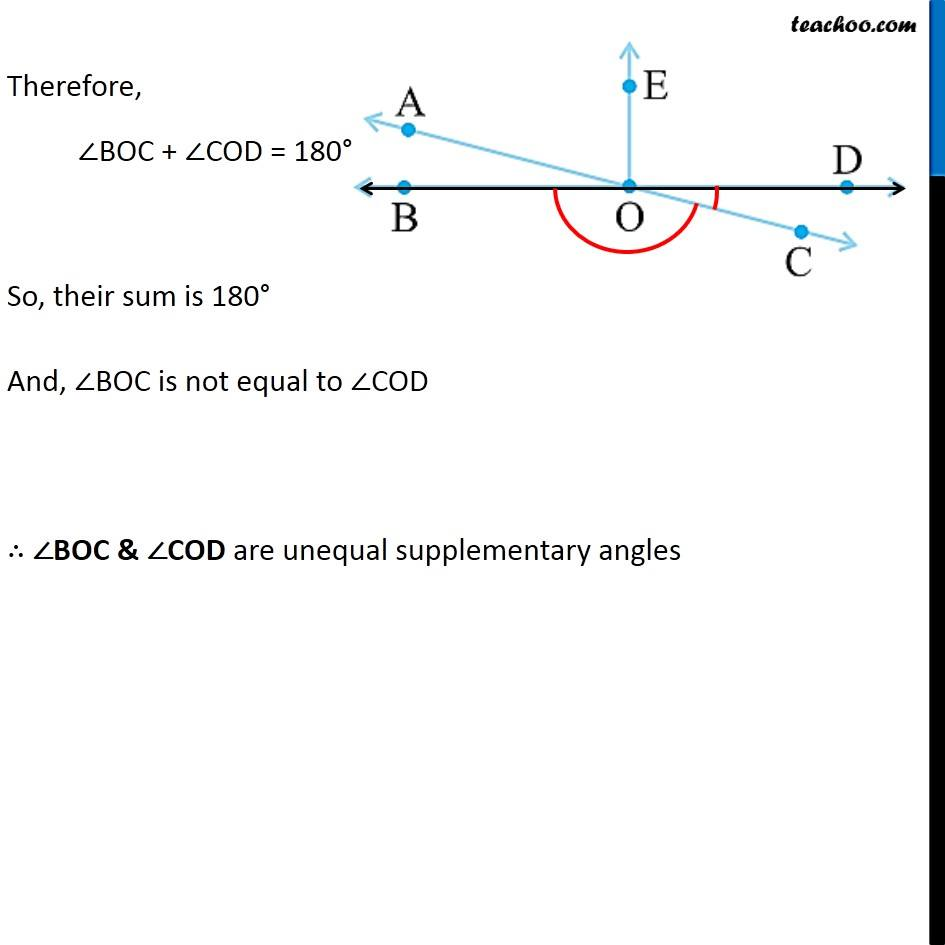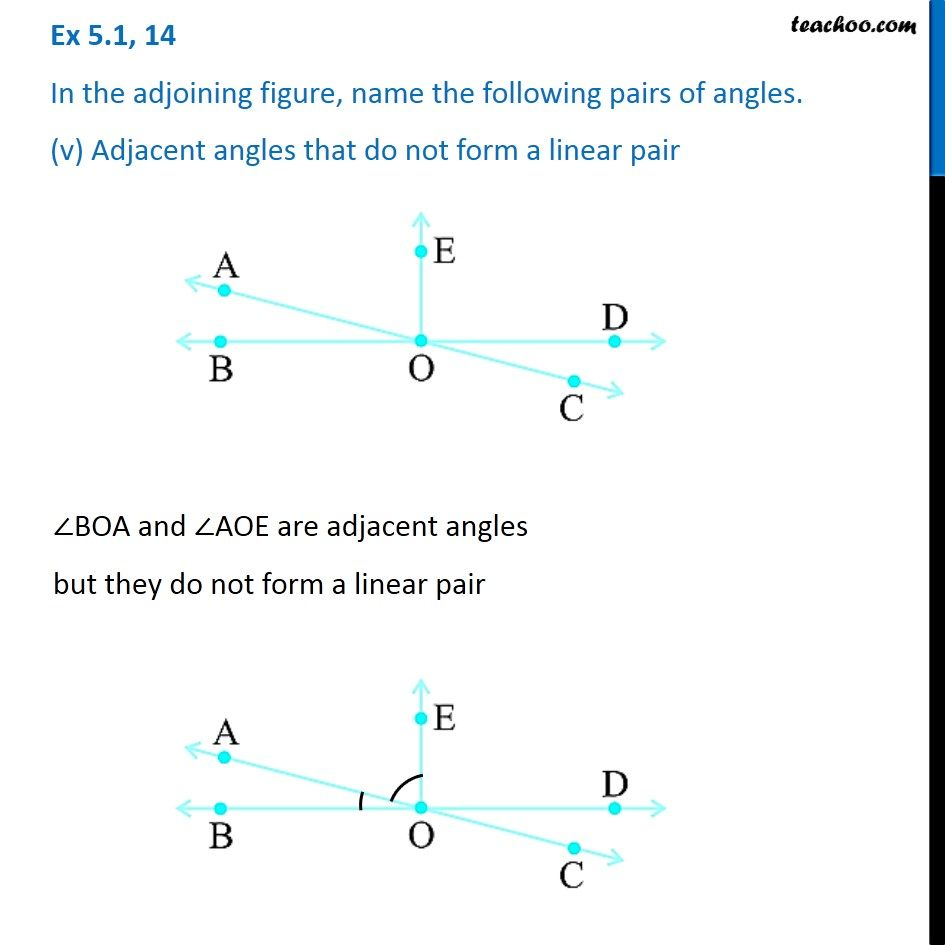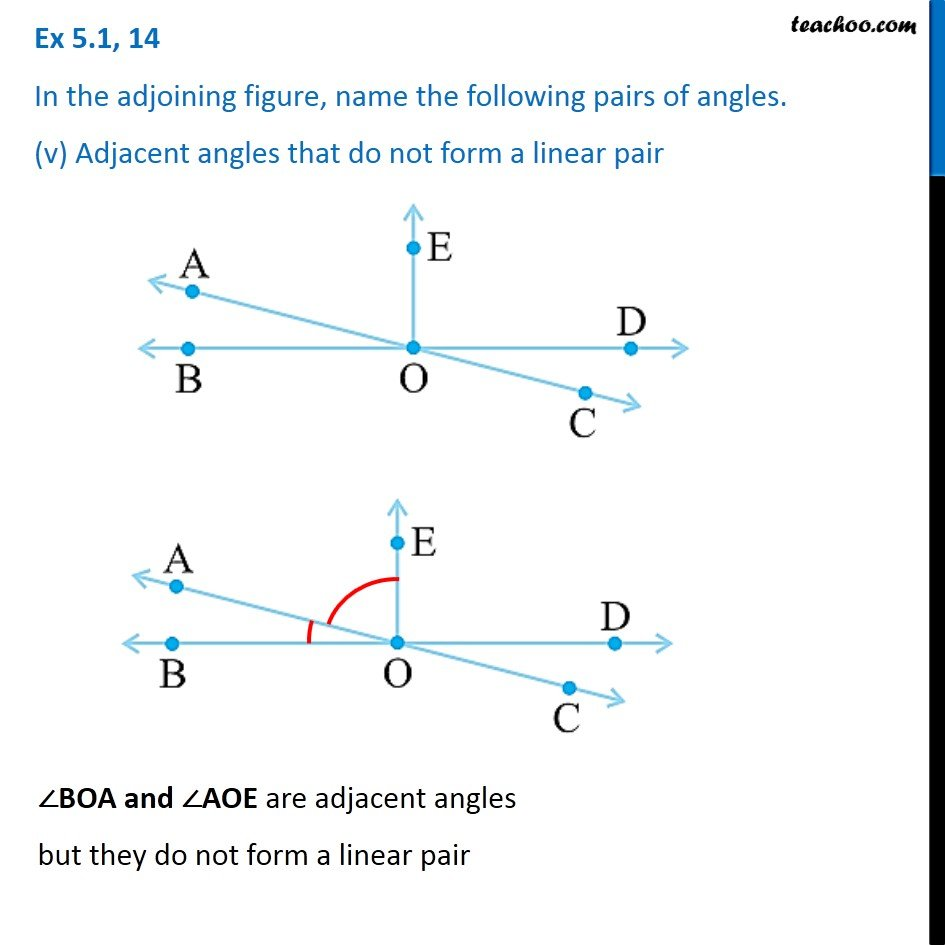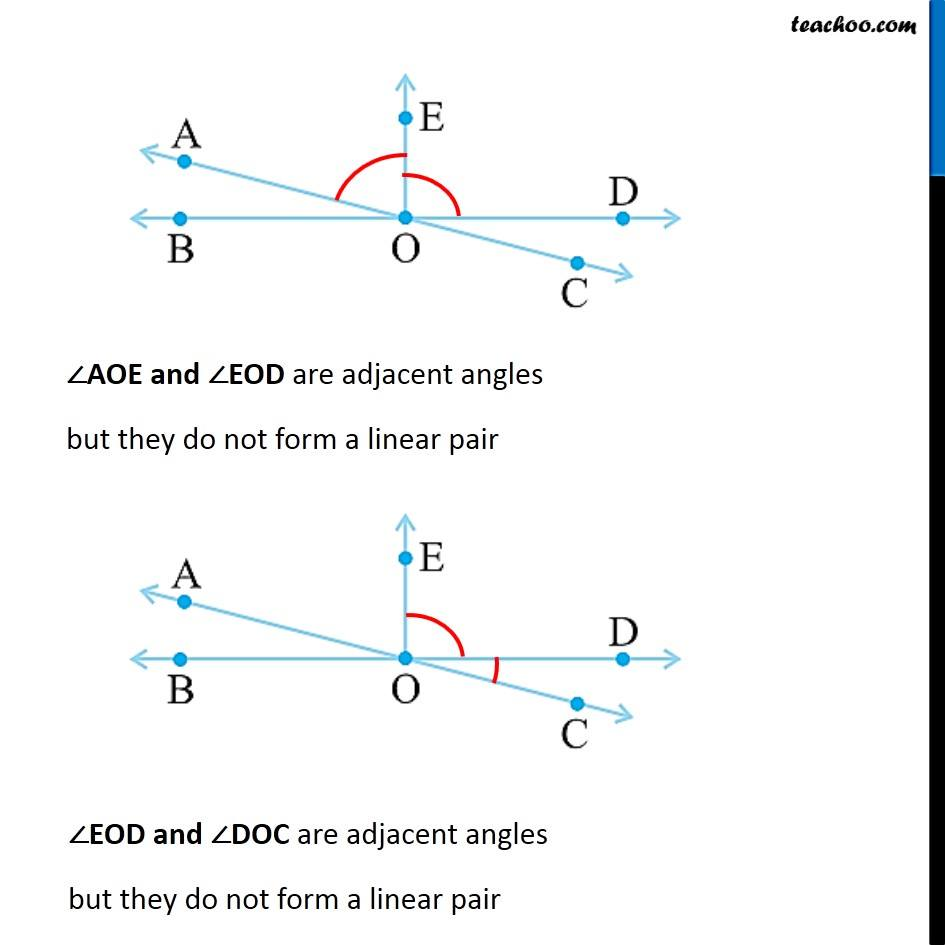1. Chapter 5 Class 7 Lines and Angles
2. Serial order wise
3. Ex 5.1

Transcript

Ex 5.1, 14 In the adjoining figure, name the following pairs of angles. (i) Obtuse vertically opposite angles∠AOD, ∠BOC are obtuse vertically opposite angles. Ex 5.1, 14 In the adjoining figure, name the following pairs of angles. (ii) Adjacent complementary angles∠BOA, ∠AOE are adjacent angles Also, ∠BOA + ∠AOE = ∠BOE ∠BOA + ∠AOE = 90° Since sum of ∠BOA & ∠AOE are 90°, they are complementary ∴ ∠BAO & ∠AOE are adjacent complementary angles Ex 5.1, 14 In the adjoining figure, name the following pairs of angles. (iii) Equal supplementary angles∠BOE and ∠EOD are equal supplementary angles i.e of 90° each. ∠BOE & ∠EOD are both 90° So, they are equal Ex 5.1, 14 In the adjoining figure, name the following pairs of angles. (iii) Equal supplementary angles ∠BOA, ∠AOE are both 90° So, they are equal And, ∠BOE + ∠EOD = 90° + 90° = 180° So, their sum is 180° ∴ They are supplementary Hence, ∠BOE & ∠EOD are equal supplementary angles Ex 5.1, 14 In the adjoining figure, name the following pairs of angles. (iv) Unequal supplementary anglesFor line BD ∠ BOC and ∠ COD are in linear pair Therefore, ∠BOC + ∠COD = 180° So, their sum is 180° And, ∠BOC is not equal to ∠COD ∴ ∠BOC & ∠COD are unequal supplementary angles Ex 5.1, 14 In the adjoining figure, name the following pairs of angles. (v) Adjacent angles that do not form a linear pair∠BOA and ∠AOE are adjacent angles but they do not form a linear pair Ex 5.1, 14 In the adjoining figure, name the following pairs of angles. (v) Adjacent angles that do not form a linear pair ∠BOA and ∠AOE are adjacent angles but they do not form a linear pair ∠AOE and ∠EOD are adjacent angles but they do not form a linear pair ∠EOD and ∠DOC are adjacent angles but they do not form a linear pair

Ex 5.1